# MAGNETIC CIRCUITS (Motors And Drives)

So far we have assumed that the source of the magnetic field is a permanent magnet. This is a convenient starting point as all of us are familiar with magnets, even if only of the fridge-door variety. But in the majority of motors, the working magnetic field is produced by coils of wire carrying current, so it is appropriate that we spend some time looking at how we arrange the coils and their associated iron ‘magnetic circuit’ so as to produce high magnetic fields which then interact with other current-carrying conductors to produce force, and hence rotation.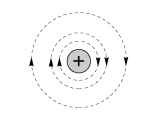Figure 1.4 Magnetic flux lines produced by a straight, current-carrying wire
First, we look at the simplest possible case of the magnetic field surrounding an isolated long straight wire carrying a steady current (Figure 1.4). (In the figure, the + sign indicates that current is flowing into the paper, while a dot is used to signify current out of the paper: these symbols can perhaps be remembered by picturing an arrow or dart, with the cross being the rear view of the letch, and the dot being the approaching point.) The flux lines form circles concentric with the wire, the field strength being greatest close to the wire. As might be expected, the field strength at any point is directly proportional to the current. The convention for determining the direction of the field is that the positive direction is taken to be the direction that a right-handed corkscrew must be rotated to move in the direction of the current.
Figure 1.4 is somewhat artificial as current can only low in a complete circuit, so there must always be a return path. If we imagine a parallel ‘go’ and ‘return’ circuit, for example, the field can be obtained by superimposing the field produced by the positive current in the go side with the field produced by the negative current in the return side, as shown in Figure 1.5.
We note how the field is increased in the region between the conductors, and reduced in the regions outside. Although Figure 1.5 strictly only applies to an infinitely long pair of straight conductors, it will probably not come as a surprise to learn that the field produced by a single turn of wire of rectangular, square or round form is very much the same as that shown in Figure 1.5. This enables us to build up a picture of the field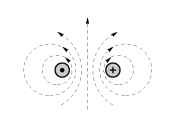Figure 1.5 Magnetic flux lines produced by current in a parallel go and return circuit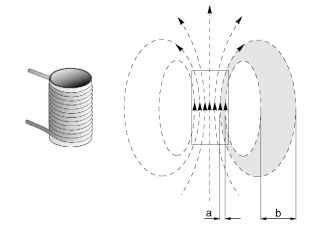Figure 1.6 Multi-turn cylindrical coil and pattern of magnetic flux produced by current in the coil. (For the sake of clarity, only the outline of the coil is shown on the right.) that would be produced in air, by the sort of coils used in motors, which typically have many turns, as shown for example in Figure 1.6.
The coil itself is shown on the left in Figure 1.6 while the flux pattern produced is shown on the right. Each turn in the coil produces a field pattern, and when all the individual field components are superimposed we see that the field inside the coil is substantially increased and that the closed flux paths closely resemble those of the bar magnet we looked at earlier. The air surrounding the sources of the field offers a homogeneous path for the flux, so once the tubes of flux escape from the concentrating influence of the source, they are free to spread out into the whole of the surrounding space. Recalling that between each pair of flux lines there is an equal amount of flux, we see that because the flux lines spread out as they leave the continues of the coil, the flux density is much lower outside than inside: for example, if the distance ‘b’ is say four times ‘a’ the flux density Bb is a quarter of Ba.
Although the flux density inside the coil is higher than outside, we would find that the flux densities which we could achieve are still too low to be of use in a motor. What is needed firstly is a way of increasing the flux density, and secondly a means for concentrating the flux and preventing it from spreading out into the surrounding space.

### Magnetomotive force (MMF)

One obvious way to increase the flux density is to increase the current in the coil, or to add more turns. We find that if we double the current, or
the number of turns, we double the total flux, thereby doubling the flux density everywhere.
We quantify the ability of the coil to produce flux in terms of its magnetomotive force (MMF). The MMF of the coil is simply the product of the number of turns (N) and the current (I), and is thus expressed in ampere-turns. A given MMF can be obtained with a large number of turns of thin wire carrying a low current, or a few turns of thick wire carrying a high current: as long as the product NI is constant, the MMF is the same.

### Electric circuit analogy

We have seen that the magnetic flux which is set up is proportional to the MMF driving it. This points to a parallel with the electric circuit, where the current (amps) that lows is proportional to the EMF (volts) driving it.
In the electric circuit, current and EMF are related by Ohm’s Law, which is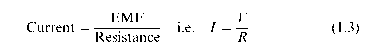For a given source EMF (volts), the current depends on the resistance of the circuit, so to obtain more current, the resistance of the circuit has to be reduced.
We can make use of an equivalent ‘magnetic Ohm’s law’ by introducing the idea of reluctance (R). The reluctance gives a measure of how difficult it is for the magnetic flux to complete its circuit, in the same way that resistance indicates how much opposition the current encounters in the electric circuit. The magnetic Ohm’s law is then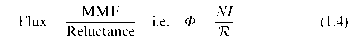We see from equation 1.4 that to increase the flux for a given MMF, we need to reduce the reluctance of the magnetic circuit. In the case of the example (Figure 1.6), this means we must replace as much as possible of the air path (which is a ‘poor’ magnetic material, and therefore constitutes a high reluctance) with a ‘good’ magnetic material, thereby reducing the reluctance and resulting in a higher flux for a given MMF.
The material which we choose is good quality magnetic steel, which for historical reasons is usually referred to as ‘iron’. This brings several very dramatic and desirable benefits, as shown in Figure 1.7.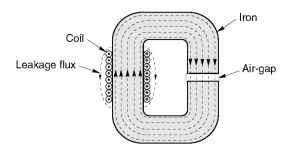Figure 1.7 Flux lines inside low-reluctance magnetic circuit with air-gap
Firstly, the reluctance of the iron paths is very much less than the air paths which they have replaced, so the total flux produced for a given MMF is very much greater. (Strictly speaking therefore, if the MMFs and cross-sections of the coils in Figures 1.6 and 1.7 are the same, many more flux lines should be shown in Figure 1.7 than in Figure 1.6, but for the sake of clarity similar numbers are indicated.) Secondly, almost all the flux is continued within the iron, rather than spreading out into the surrounding air. We can therefore shape the iron parts of the magnetic circuit as shown in Figure 1.7 in order to guide the flux to wherever it is needed. And finally, we see that inside the iron, the flux density remains uniform over the whole cross-section, there being so little reluctance that there is no noticeable tendency for the flux to crowd to one side or another.
Before moving on to the matter of the air-gap, we should note that a question which is often asked is whether it is important for the coils to be wound tightly onto the magnetic circuit, and whether, if there is a multi-layer winding, the outer turns are as effective as the inner ones. The answer, happily, is that the total MMF is determined solely by the number of turns and the current, and therefore every complete turn makes the same contribution to the total MMF, regardless of whether it happens to be tightly or loosely wound. Of course it does make sense for the coils to be wound as tightly as is practicable, since this not only minimises the resistance of the coil (and thereby reduces the heat loss) but also makes it easier for the heat generated to be conducted away to the frame of the machine.

### The air-gap

In motors, we intend to use the high flux density to develop force on current-carrying conductors. We have now seen how to create a high flux density inside the iron parts of a magnetic circuit, but, of course, it is
physically impossible to put current-carrying conductors inside the iron. We therefore arrange for an air-gap in the magnetic circuit, as shown in Figure 1.7. We will see shortly that the conductors on which the force is to be produced will be placed in this air-gap region.
If the air-gap is relatively small, as in motors, we find that the flux jumps across the air-gap as shown in Figure 1.7, with very little tendency to balloon out into the surrounding air. With most of the flux lines going straight across the air-gap, the lux density in the gap region has the same high value as it does inside the iron.
In the majority of magnetic circuits consisting of iron parts and one or more air-gaps, the reluctance of the iron parts is very much less than the reluctance of the gaps. At first sight this can seem surprising, since the distance across the gap is so much less than the rest of the path through the iron. The fact that the air-gap dominates the reluctance is simply a reflection of how poor air is as a magnetic medium, compared to iron. To put the comparison in perspective, if we calculate the reluctances of two paths of equal length and cross-sectional area, one being in iron and the other in air, the reluctance of the air path will typically be 1000 times greater than the reluctance of the iron path. (The calculation of reluctance will be discussed in Section 1.3.4.)
Returning to the analogy with the electric circuit, the role of the iron parts of the magnetic circuit can be likened to that of the copper wires in the electric circuit. Both offer little opposition to low (so that a negligible fraction of the driving force (MMF or EMF) is wasted in conveying the low to where it is usefully exploited) and both can be shaped to guide the low to its destination. There is one important difference, however. In the electric circuit, no current will low until the circuit is completed, after which all the current is continued inside the wires. With an iron magnetic circuit, some flux can low (in the surrounding air) even before the iron is installed. And although most of the flux will subsequently take the easy route through the iron, some will still leak into the air, as shown in Figure 1.7. We will not pursue leakage flux here, though it is sometimes important, as will be seen later.

### Reluctance and air-gap flux densities

If we neglect the reluctance of the iron parts of a magnetic circuit, it is easy to estimate the flux density in the air-gap. Since the iron parts are then in effect ‘perfect conductors’ of flux, none of the source MMF (NI) is used in driving the flux through the iron parts, and all of it is available to push the flux across the air-gap. The situation depicted in Figure 1.7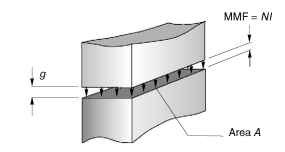Figure 1.8 Air-gap region, with MMF acting across opposing pole-faces therefore reduces to that shown in Figure 1.8, where an MMF of NI is applied directly across an air-gap of length.
To determine how much flux will cross the gap, we need to know its reluctance. As might be expected, the reluctance of any part of the magnetic circuit depends on its dimensions, and on its magnetic properties, and the reluctance of a rectangular ‘prism’ of air, of cross-sectional area A and length g as in Figure 1.8 is given by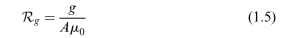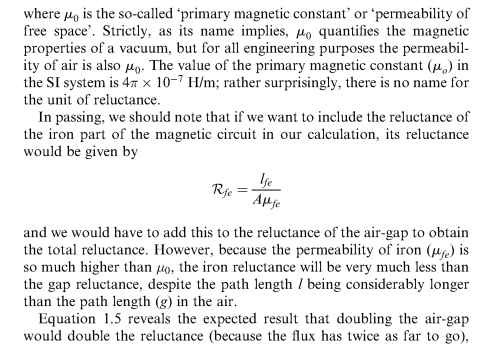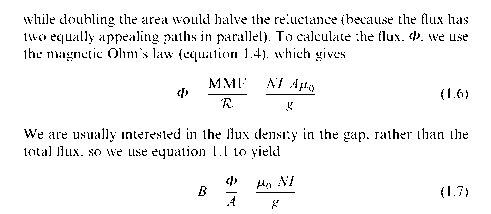Equation 1.7 is delightfully simple, and from it we can calculate the air-gap flux density once we know the MMF of the coil (NI) and the length of the gap (g). We do not need to know the details of the coil-winding as long as we know the product of the turns and the current, nor do we need to know the cross-sectional area of the magnetic circuit in order to obtain the flux density (though we do if we want to know the total flux, see equation 1.6).
For example, suppose the magnetising coil has 250 turns, the current is 2 A, and the gap is 1 mm. The flux density is then given by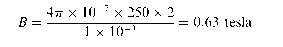(We could of course obtain the same result using an exciting coil of 50 turns carrying a current of 10 A, or any other combination of turns and current giving an MMF of 500 ampere-turns.)
If the cross-sectional area of the iron was constant at all points, the flux density would be 0.63 T everywhere. Sometimes, as has already been mentioned, the cross-section of the iron reduces at points away from the air-gap, as shown for example in Figure 1.3. Because the flux is compressed in the narrower sections, the flux density is higher, and in Figure 1.3 if the flux density at the air-gap and in the adjacent pole-faces is once again taken to be 0.63 T, then at the section aa’ (where the area is only half that at the air-gap) the flux density will be 2 x 0.63 = 1.26 T.

### Saturation

It would be reasonable to ask whether there is any limit to the lux density at which the iron can be operated. We can anticipate that there must be a limit, or else it would be possible to squash the lux into a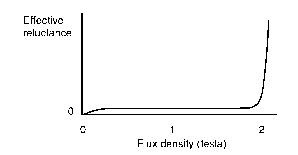Figure 1.9 Sketch showing how the effective reluctance of iron increases rapidly as the flux density approaches saturation vanishingly small cross-section, which we know from experience is not the case. In fact there is a limit, though not a very sharply denied one.
Earlier we noted that the iron has almost no reluctance, at least not in comparison with air. Unfortunately this happy state of affairs is only true as long as the flux density remains below about 1.6 – 1.8 T, depending on the particular steel in question. If we try to work the iron at higher flux densities, it begins to exhibit significant reluctance, and no longer behaves like an ideal conductor of flux. At these higher flux densities, a significant proportion of the source MMF is used in driving the flux through the iron. This situation is obviously undesirable, since less MMF remains to drive the flux across the air-gap. So just as we would not recommend the use of high-resistance supply leads to the load in an electric circuit, we must avoid overloading the iron parts of the magnetic circuit.
The emergence of significant reluctance as the flux density is raised is illustrated qualitatively in Figure 1.9.
When the reluctance begins to be appreciable, the iron is said to be beginning to ‘saturate’. The term is apt, because if we continue increasing the MMF, or reducing the area of the iron, we will eventually reach an almost constant flux density, typically around 2 T. To avoid the undesirable effects of saturation, the size of the iron parts of the magnetic circuit are usually chosen so that the flux density does not exceed about 1.5 T. At this level of flux density, the reluctance of the iron parts will be small in comparison with the air-gap.

### Magnetic circuits in motors

The reader may be wondering why so much attention has been focused on the gapped C-core magnetic circuit, when it appears to bear little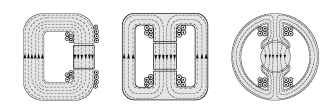Figure 1.10 Evolution of d.c. motor magnetic circuit from gapped C-core
resemblance to the magnetic circuits found in motors. We will now see that it is actually a short step from the C-core to a magnetic motor circuit, and that no fundamentally new ideas are involved.
The evolution from C-core to motor geometry is shown in Figure 1.10, which should be largely self-explanatory, and relates to the field system of a d.c. motor.
We note that the first stage of evolution (Figure 1.10, left) results in the original single gap of length g being split into two gaps of length g/2, reflecting the requirement for the rotor to be able to turn. At the same time the single magnetising coil is split into two to preserve symmetry. (Relocating the magnetising coil at a different position around the magnetic circuit is of course in order, just as a battery can be placed anywhere in an electric circuit.) Next, (Figure 1.10, centre) the single magnetic path is split into two parallel paths of half the original cross-section, each of which carries half of the flux: and finally (Figure 1.10, right), the flux paths and pole-faces are curved to match the rotor. The coil now has several layers in order to it the available space, but as discussed earlier this has no adverse effect on the MMF. The air-gap is still small, so the flux crosses radially to the rotor.

Next post:

Previous post: